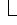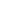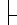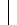# DataLabel Object

ChartSeriesCollection (Series)DataLabels (DataLabel)Points (Point)DataLabelTrendlines (Trendline)DataLabel

Represents the data label for the specified point or trendline in a chart. For a series, the DataLabel object is a member of the DataLabels collection, which contains a DataLabel object for each point. For a series without definable points (such as an area series), the DataLabels collection contains a single DataLabel object.

## Using the DataLabel Object

Use DataLabels(index), where index is the data label's index number, to return a single DataLabel object. The following example sets the number format for the fifth data label in series one in the chart.

``myChart.SeriesCollection(1).DataLabels(5).NumberFormat = "0.000"``

Use the DataLabel property to return the DataLabel object for a single point. The following example turns on the data label for the second point in series one in the chart, and sets the data label text to "Saturday."

``````With myChart
With .SeriesCollection(1).Points(2)
.HasDataLabel = True
.DataLabel.Text = "Saturday"
End With
End With``````

For a trendline, the DataLabel property returns the text shown with the trendline. This can be the equation, the R-squared value, or both (if both are showing). The following example sets the trendline text to show only the equation and then places the data label text in cell A1 on the datasheet.

``````With myChart.SeriesCollection(1).Trendlines(1)
.DisplayRSquared = False
.DisplayEquation = True
x = .DataLabel.Text
End With
With myChart.Application.DataSheet
.Range("A1").Value = x
End With``````